# Skewness

Assistant Professor em IAMR Group Of institutions
18 de Oct de 2014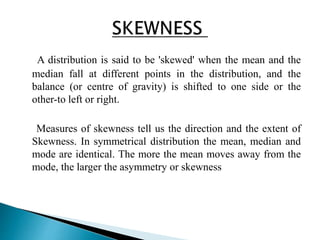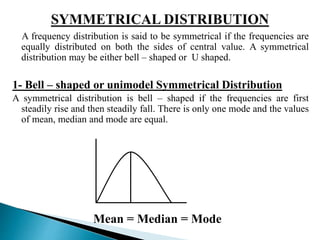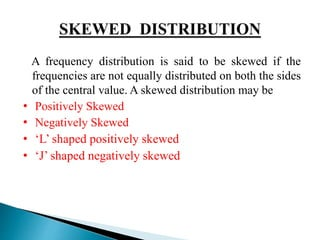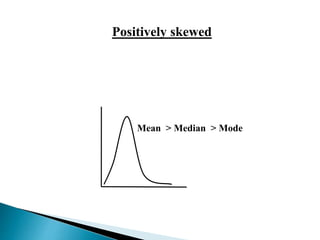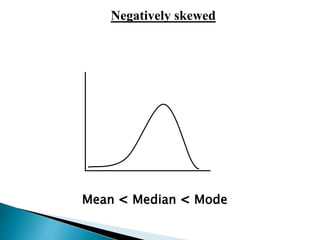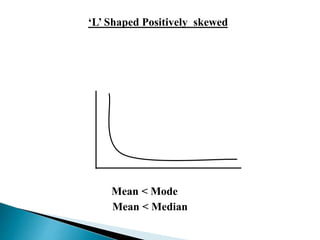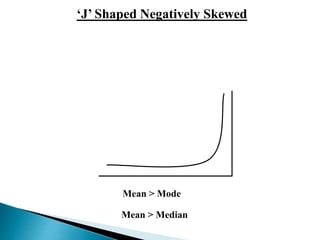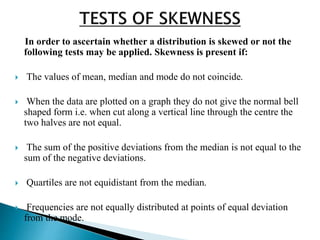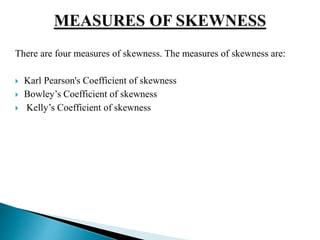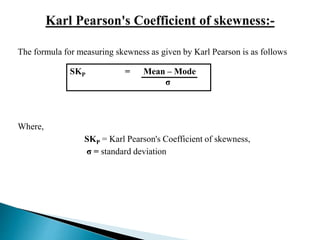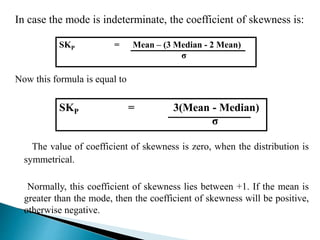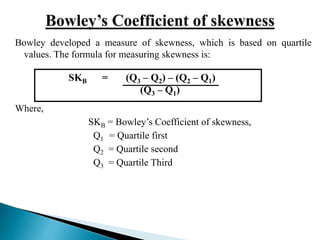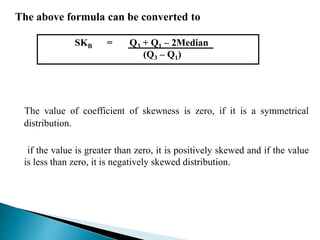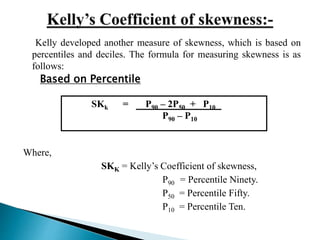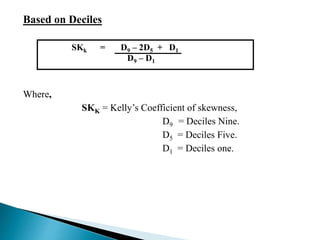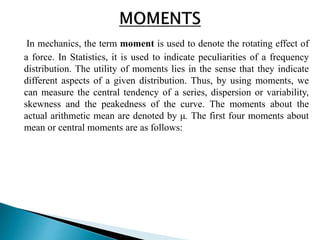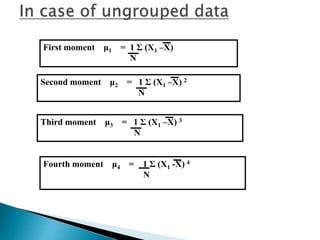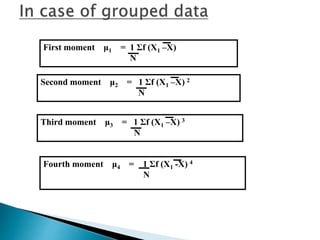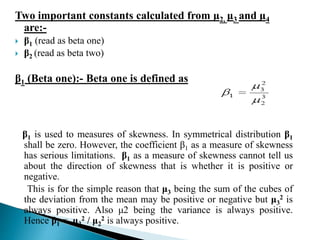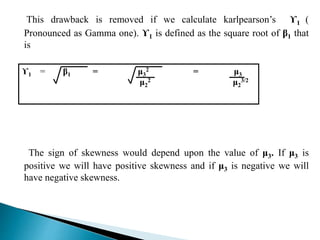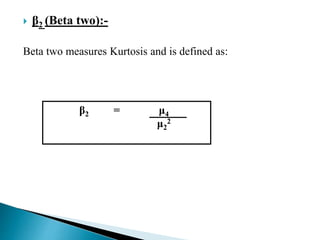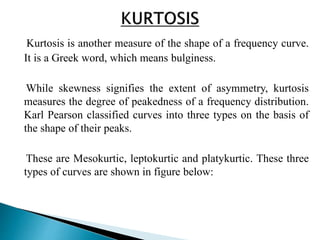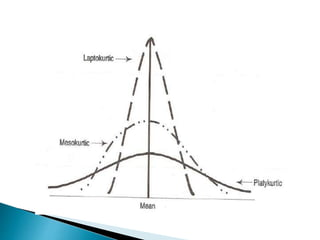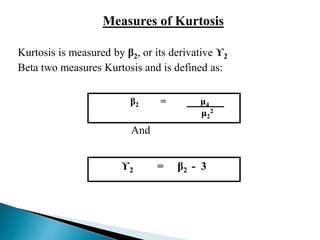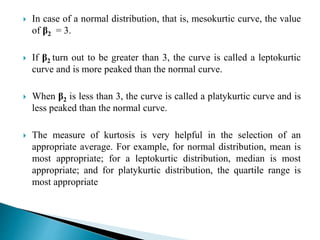1 de 27

### Skewness

• 1. Prof. Rajkumar Teotia Institute of Advanced Management and Research (IAMR) Address: 9th Km Stone, NH-58, Delhi-Meerut Road, Duhai,Ghaziabad (U.P) - 201206 Ph:0120-2675904/905 Mob:9999052997 Fax: 0120-2679145 e mail: rajkumarteotia@iamrindia.com
• 3. A distribution is said to be 'skewed' when the mean and the median fall at different points in the distribution, and the balance (or centre of gravity) is shifted to one side or the other-to left or right. Measures of skewness tell us the direction and the extent of Skewness. In symmetrical distribution the mean, median and mode are identical. The more the mean moves away from the mode, the larger the asymmetry or skewness
• 4. A frequency distribution is said to be symmetrical if the frequencies are equally distributed on both the sides of central value. A symmetrical distribution may be either bell – shaped or U shaped. 1- Bell – shaped or unimodel Symmetrical Distribution A symmetrical distribution is bell – shaped if the frequencies are first steadily rise and then steadily fall. There is only one mode and the values of mean, median and mode are equal. Mean = Median = Mode
• 5. A frequency distribution is said to be skewed if the frequencies are not equally distributed on both the sides of the central value. A skewed distribution may be • Positively Skewed • Negatively Skewed • ‘L’ shaped positively skewed • ‘J’ shaped negatively skewed
• 6. Positively skewed Mean ˃ Median ˃ Mode
• 7. Negatively skewed Mean ˂ Median ˂ Mode
• 8. ‘L’ Shaped Positively skewed Mean ˂ Mode Mean ˂ Median
• 9. ‘J’ Shaped Negatively Skewed Mean ˃ Mode Mean ˃ Median
• 10. In order to ascertain whether a distribution is skewed or not the following tests may be applied. Skewness is present if:  The values of mean, median and mode do not coincide.  When the data are plotted on a graph they do not give the normal bell shaped form i.e. when cut along a vertical line through the centre the two halves are not equal.  The sum of the positive deviations from the median is not equal to the sum of the negative deviations.  Quartiles are not equidistant from the median.  Frequencies are not equally distributed at points of equal deviation from the mode.
• 11. There are four measures of skewness. The measures of skewness are:  Karl Pearson's Coefficient of skewness  Bowley’s Coefficient of skewness  Kelly’s Coefficient of skewness
• 12. The formula for measuring skewness as given by Karl Pearson is as follows Where, SKP = Mean – Mode σ SKP = Karl Pearson's Coefficient of skewness, σ = standard deviation
• 13. In case the mode is indeterminate, the coefficient of skewness is: SKP = Mean – (3 Median - 2 Mean) Now this formula is equal to σ SKP = 3(Mean - Median) σ The value of coefficient of skewness is zero, when the distribution is symmetrical. Normally, this coefficient of skewness lies between +1. If the mean is greater than the mode, then the coefficient of skewness will be positive, otherwise negative.
• 14. Bowley developed a measure of skewness, which is based on quartile values. The formula for measuring skewness is: Where, SKB = (Q3 – Q2) – (Q2 – Q1) (Q3 – Q1) SKB = Bowley’s Coefficient of skewness, Q1 = Quartile first Q2 = Quartile second Q3 = Quartile Third
• 15. The above formula can be converted to SKB = Q3 + Q1 – 2Median (Q3 – Q1) The value of coefficient of skewness is zero, if it is a symmetrical distribution. if the value is greater than zero, it is positively skewed and if the value is less than zero, it is negatively skewed distribution.
• 16. Kelly developed another measure of skewness, which is based on percentiles and deciles. The formula for measuring skewness is as follows: Based on Percentile Where, SKk = P90 – 2P50 + P10 P90 – P10 SKK = Kelly’s Coefficient of skewness, P90 = Percentile Ninety. P50 = Percentile Fifty. P10 = Percentile Ten.
• 17. Based on Deciles Where, SKk = D9 – 2D5 + D1 SKK = Kelly’s Coefficient of skewness, D9 = Deciles Nine. D5 = Deciles Five. D1 = Deciles one. D9 – D1
• 18. In mechanics, the term moment is used to denote the rotating effect of a force. In Statistics, it is used to indicate peculiarities of a frequency distribution. The utility of moments lies in the sense that they indicate different aspects of a given distribution. Thus, by using moments, we can measure the central tendency of a series, dispersion or variability, skewness and the peakedness of the curve. The moments about the actual arithmetic mean are denoted by μ. The first four moments about mean or central moments are as follows:
• 19. First moment μ1 = 1 Σ (X1 –X) N Second moment μ2 = 1 Σ (X1 –X) 2 N Third moment μ3 = 1 Σ (X1 –X) 3 N Fourth moment μ4 = 1 Σ (X1 -X) 4 N
• 20. First moment μ1 = 1 Σf (X1 –X) N Second moment μ2 = 1 Σf (X1 –X) 2 N Third moment μ3 = 1 Σf (X1 –X) 3 N Fourth moment μ4 = 1 Σf (X1 -X) 4 N
• 21. Two important constants calculated from μ2, μ3 and μ4 are:-  β1 (read as beta one)  β2 (read as beta two) β1 (Beta one):- Beta one is defined as βis used to measures of skewness. In symmetrical distribution β1 1 shall be zero. However, the coefficient βas a measure of skewness 1 has serious limitations. βas a measure of skewness cannot tell us 1 about the direction of skewness that is whether it is positive or negative. This is for the simple reason that μbeing the sum of the cubes of 3 the deviation from the mean may be positive or negative but μ2 is 3 always positive. Also μ2 being the variance is always positive. Hence β= μ2 / μ2 is always positive. 1 3 2
• 22. This drawback is removed if we calculate karlpearson’s ϒ1 ( Pronounced as Gamma one). ϒ1 is defined as the square root of β1 that is 2 = μ3 μ2 ϒ1 = β1 = μ3 2 μ2 3/2 The sign of skewness would depend upon the value of μ3. If μ3 is positive we will have positive skewness and if μ3 is negative we will have negative skewness.
• 23.  β2 (Beta two):- Beta two measures Kurtosis and is defined as: β2 = μ4 μ2 2
• 24. Kurtosis is another measure of the shape of a frequency curve. It is a Greek word, which means bulginess. While skewness signifies the extent of asymmetry, kurtosis measures the degree of peakedness of a frequency distribution. Karl Pearson classified curves into three types on the basis of the shape of their peaks. These are Mesokurtic, leptokurtic and platykurtic. These three types of curves are shown in figure below:
• 26. Measures of Kurtosis Kurtosis is measured by β2, or its derivative ϒ2 Beta two measures Kurtosis and is defined as: β2 = μ4 And μ2 2 ϒ2 = β2 - 3
• 27.  In case of a normal distribution, that is, mesokurtic curve, the value of β2 = 3.  If β2 turn out to be greater than 3, the curve is called a leptokurtic curve and is more peaked than the normal curve.  When β2 is less than 3, the curve is called a platykurtic curve and is less peaked than the normal curve.  The measure of kurtosis is very helpful in the selection of an appropriate average. For example, for normal distribution, mean is most appropriate; for a leptokurtic distribution, median is most appropriate; and for platykurtic distribution, the quartile range is most appropriate